Probability_and_StatisticsHome   Algebra   Geometry   Arithmetic   Trigonometry   Probability/Statistics   Calculus   Mechanics GED   SAT   ACT   College Mathematics   GRE   School Supplies   About Us

Probability & Statistics

 Electronic Textbooks TDownloads - Preview, Get, Practice!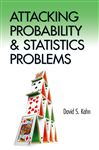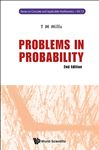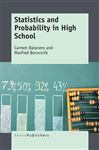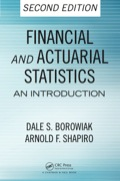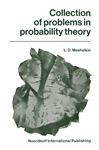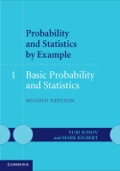Paperback Textbooks (New and Used)Examples
 Probability: A coin is tossed twice.   (a) Indicate a sample space for this experiment.   (b) Indicate also a sample space for the number of heads obtained.  Solutions    (a) A coin has two sides, heads and tails. If the coin is tossed once, then either H (heads) or T (tails) could occur. If it is tossed        twice, both heads could occur. Both tails could occur also. Likewise, either heads or tails could occur on either toss. The        appropriate sample space S for this experiment would be the set of possible outcomes, being S = {TT, HH, TH, HT}. Both        letters together represent the possibilities of the first and second toss.   (b) For the number of heads, there could be 1 (one) on the first toss and 1 on the second, making it 2 heads. There could also be        1 on the first and 0 (none) on the second. Vice versa, there could be 0 on the first and 1 on the second. There could even be 0        on both tosses. The sample space then for this would be S = {2, 1, 0}.for Math Practice, GED  SAT  ACT  College Math  GRE

 More School Supplies and other useful items *  * *